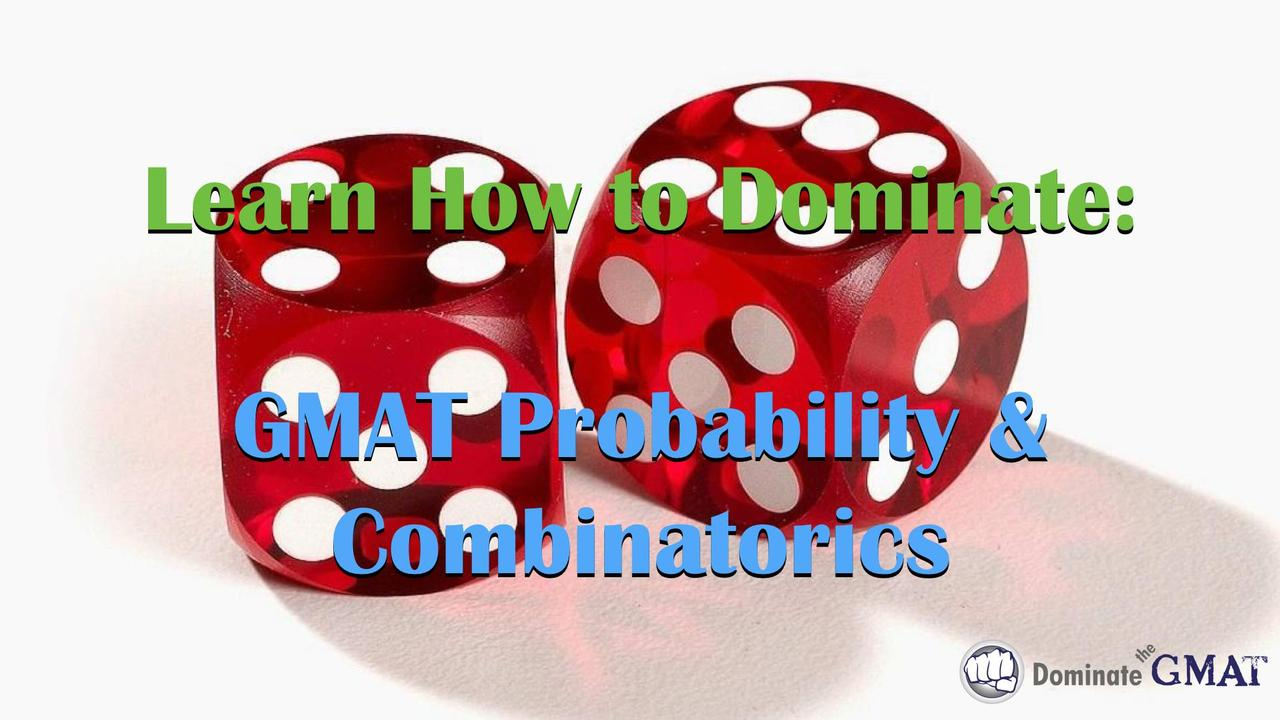# GMAT Probability & Combinatorics - Video Course

One of the more challenging subsets of questions tested on the GMAT quantitative section involves probability and combinatorics (combinations and permutations), and they’re tested in both Problem Solving and Data Sufficiency question formats.

Fortunately, there are a few rules that, once learned, make GMAT probability questions much easier to master. Likewise, you don’t need to memorize complex combinations and permutations formulas (as taught in most GMAT textbooks and prep courses) to solve the types of “How many ways…?” questions tested on the GMAT. This course will teach you a more effective strategy for tackling these types of combination questions, as well as all types of probability questions, saving you precious time and enabling you to get more right answers on test day.

#### Specifically, the GMAT video tutorials in this module cover:

• A detailed overview of GMAT probability questions;
• Core probability rules that will enable you to solve every type of GMAT probability problem;
• Independent and conditional probability outcomes;
• Understanding how “and” and “or” translate into mathematical notation, and how to solve questions involving those variations;
• The “Fundamental Principle of Counting” and how it applies to GMAT “counting” questions;
• Two methodologies for solving “How many ways…?” problems;
• Combinations and Permutations;
• Numerous application examples to fully illustrate all of the concepts taught in this course;
• And more!

Oh, and did we mention that this course includes a practice worksheet that will give you the opportunity to apply what you learn to real sample GMAT probability and combinatorics questions? Each question of the worksheet comes with a detailed video answer explanation showing you the most efficient way to solve it.

So what are you waiting for? Take your GMAT preparation to the next level. Add this course to your library now and prepare to dominate the GMAT!